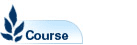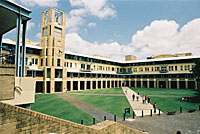Discrete Mathematics - MATH1081

Faculty: Faculty of Science

Course Outline: http://www.maths.unsw.edu.au/

Campus: Sydney

Units of Credit: 6

Indicative Contact Hours per Week: 6

Enrolment Requirements:

Corequisite: MATH1131 or MATH1141 or MATH1151.

Excluded: MATH1090

Tuition Fee: See Tuition Fee Schedule

Further Information: See Class Timetable

View course information for previous years.

Description

Role of proof in mathematics, logical reasoning and implication, different types of proofs. Sets, algebra of sets, operations on sets. Mathematical logic, truth tables, syntax, induction. Graphs and directed graphs, basic graph algorithms. Counting, combinatorial identities, binomial and multinomial theorems. Binary operations and their properties, ordered structures. Recursion relations.

Assumed knowledge: HSC Mathematics Extension 1. Students will be expected to have achieved a combined mark of at least 100 in Mathematics and Mathematics Extension 1.